# DFA for exactly one of a and at least one of b

Deterministic Finite Automata (DFA) is defined as an abstract mathematical concept which is used to solve various specific problems in different software and hardware.In this type of problems we have some given perimeters according to which we should design DFA.

• DFA should have one of a
• DFA should have at least one b

This DFA should accept the strings such as ab, ba, abb, bab, bba, abbb, babb, bbab, bbba, abbbb…. etc but it should not accept string such as a, b, bb, bbb, aabb, ababa… etc.

Designing step-by-step :

Step-1:
Take a initial state A,and smallest possible string are ab and ba, if A takes ‘a’ as first input alphabet it goes to state B and if A takes ‘b’ as first input alphabet it goes to state C.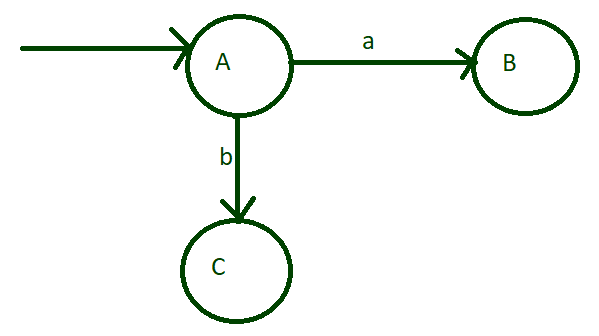Step-2:
Now think about state B, if it takes input alphabet ‘a’,it breaks our condition of only one ‘a’ but if takes input alphabet ‘b’ it makes a acceptable string and now it goes to state D which is set to final state.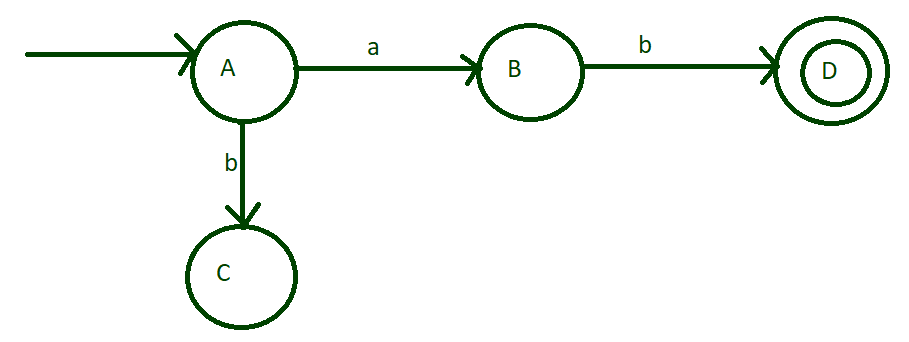Step-3:
On state C if it can take any numbers of ‘b’ which is possible and it can also take ‘a’ as input alphabet.On alphabet ‘b’ it remains on same state but on input ‘a’ it goes to state E which will set to final state.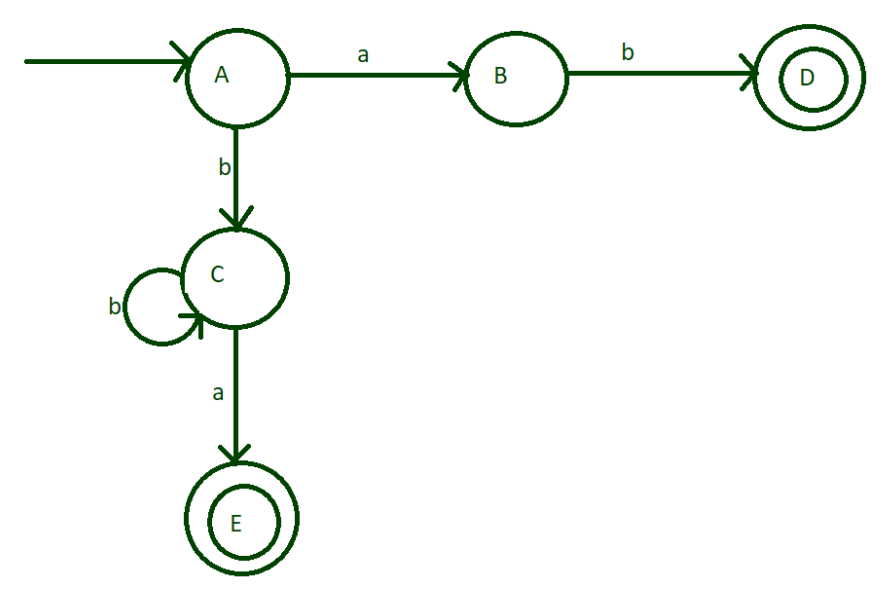Step-4:
Input alphabet ‘a’ of state B breaks the condition so it goes to some dead state(Q).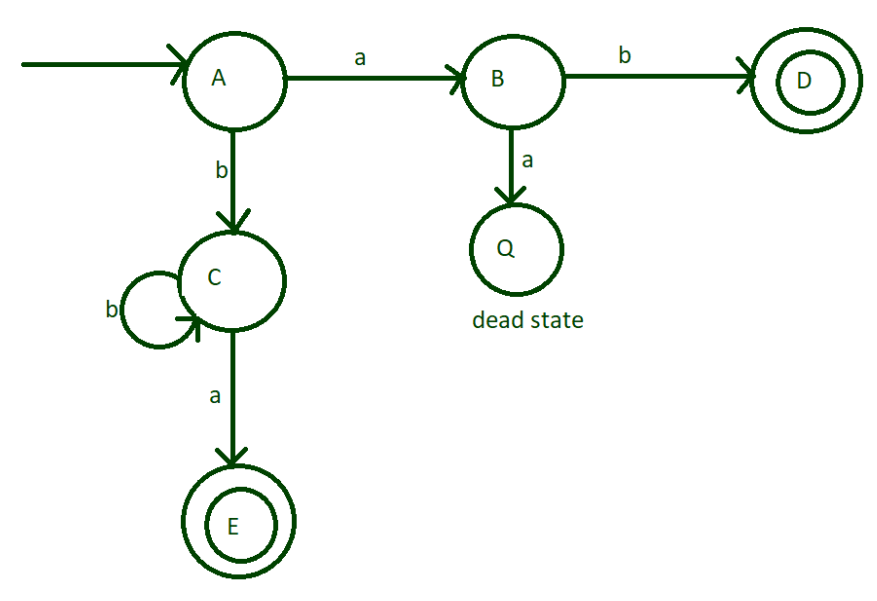Step-5:
Till now, our machine accepts string which are end from ‘a’ and ‘ab’.But what about if ‘a’ comes in middle such as bab,babb,bbab etc and what if there are many ‘b’ in the end.To do that give self loop of ‘b’ to both final stat and send their ‘a’ to dead state.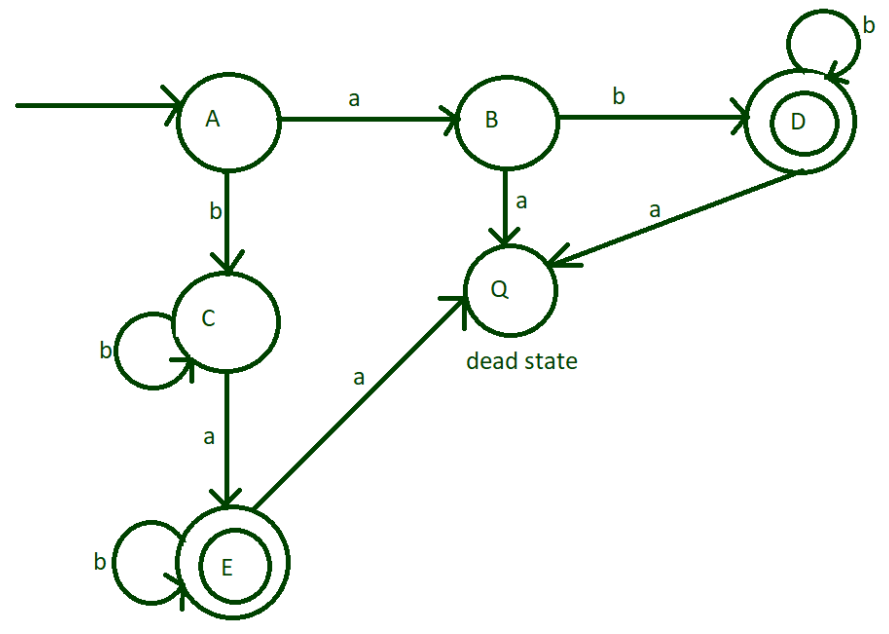Note –
Input alphabets of dead state is going to dead state only that is why these are not showing in diagrams.

Transition Table and Transition Rules of above diagram.

`finite set of states = {A, B, C, D, E, Q} `

In transition table initial state is represented by → and the final state are E and D.

`set of input alphabets = {a, b} `

STATE INPUT (a) INPUT (b)
→A B C
C E C